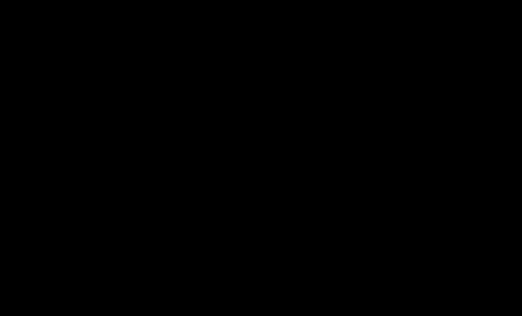# Draw Rainbow using Turtle Graphics in Python

Turtle is an inbuilt module in Python. It provides:

1. Drawing using a screen (cardboard).
2. Turtle (pen).

To draw something on the screen, we need to move the turtle (pen), and to move the turtle, there are some functions like the forward(), backward(), etc.

Prerequisite: Turtle Programming Basics

### Draw Rainbow Using Turtle Graphics

In this section, we will discuss how to draw a Rainbow using two different ways using Turtle Graphics.

Approach:

1. Import Turtle.
2. Set screen
3. Make Turtle Object
4. Define colors used for drawing
5. Loop to draw semi-circles oriented by 180-degree position.

Example 1:

## python3

 `# Import turtle package` `import` `turtle`   `# Creating a turtle screen object` `sc ``=` `turtle.Screen()`   `# Creating a turtle object(pen)` `pen ``=` `turtle.Turtle()`   `# Defining a method to form a semicircle` `# with a dynamic radius and color` `def` `semi_circle(col, rad, val):`   `    ``# Set the fill color of the semicircle` `    ``pen.color(col)`   `    ``# Draw a circle` `    ``pen.circle(rad, ``-``180``)`   `    ``# Move the turtle to air` `    ``pen.up()`   `    ``# Move the turtle to a given position` `    ``pen.setpos(val, ``0``)`   `    ``# Move the turtle to the ground` `    ``pen.down()`   `    ``pen.right(``180``)`     `# Set the colors for drawing` `col ``=` `[``'violet'``, ``'indigo'``, ``'blue'``, ` `       ``'green'``, ``'yellow'``, ``'orange'``, ``'red'``]`   `# Setup the screen features` `sc.setup(``600``, ``600``)`   `# Set the screen color to black` `sc.bgcolor(``'black'``)`   `# Setup the turtle features` `pen.right(``90``)` `pen.width(``10``)` `pen.speed(``7``)`   `# Loop to draw 7 semicircles` `for` `i ``in` `range``(``7``):` `    ``semi_circle(col[i], ``10``*``(` `      ``i ``+` `8``), ``-``10``*``(i ``+` `1``))`   `# Hide the turtle` `pen.hideturtle()`

Output:Example 2:

## Python3

 `import` `turtle`   `mypen``=` `turtle.Turtle()` `mypen.shape(``'turtle'``)` `mypen.speed(``10``)`   `window``=` `turtle.Screen()` `window.bgcolor(``'white'``)` `rainbow``=` `[``'red'``,``'orange'``,``'yellow'``,``'green'``,``'blue'``,``'indigo'``,``'violet'``]` `size``=` `180` `mypen.penup()` `mypen.goto(``0``,``-``180``)` `for` `color ``in` `rainbow:` `    ``mypen.color(color)` `    ``mypen.fillcolor(color)` `    ``mypen.begin_fill()` `    ``mypen.circle(size)` `    ``mypen.end_fill()` `    ``size``-``=``20`   `turtle.done()`

Output:

Whether you're preparing for your first job interview or aiming to upskill in this ever-evolving tech landscape, GeeksforGeeks Courses are your key to success. We provide top-quality content at affordable prices, all geared towards accelerating your growth in a time-bound manner. Join the millions we've already empowered, and we're here to do the same for you. Don't miss out - check it out now!

Previous
Next